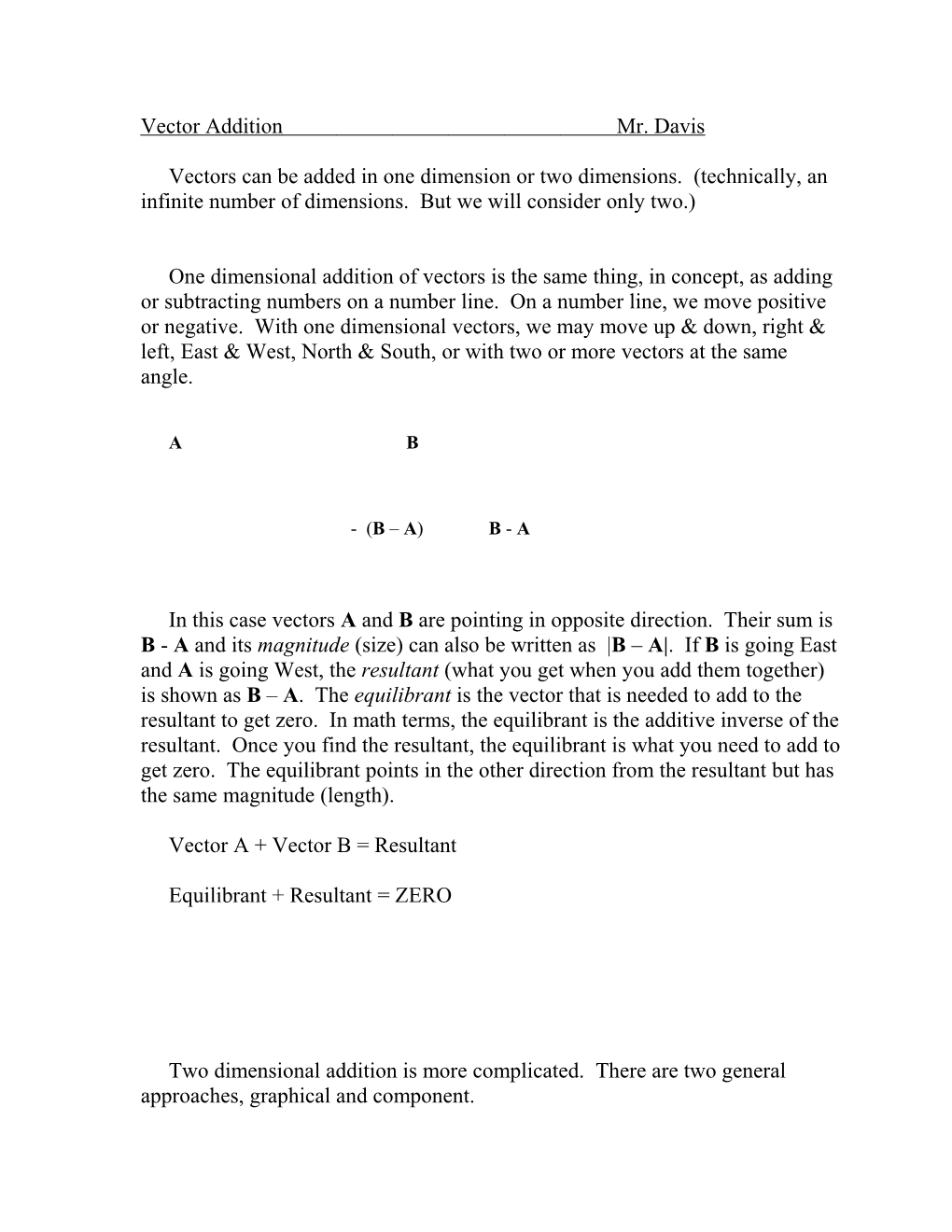Vectors can be added in one dimension or two dimensions. (technically, an infinite number of dimensions. But we will consider only two.)

One dimensional addition of vectors is the same thing, in concept, as adding or subtracting numbers on a number line. On a number line, we move positive or negative. With one dimensional vectors, we may move up & down, right left, East & West, North & South, or with two or more vectors at the same angle.

A B

- (B – A) B - A

In this case vectors A and B are pointing in opposite direction. Their sum is B - Aand its magnitude (size) can also be written as |B – A|. If B is going East and A is going West, the resultant (what you get when you add them together) is shown as B – A. The equilibrant isthe vector that is needed to add to the resultant to get zero. In math terms, the equilibrant is the additive inverse of the resultant. Once you find the resultant, the equilibrant is what you need to add to get zero. The equilibrant points in the other direction from the resultant but has the same magnitude (length).

Vector A + Vector B = Resultant

Equilibrant + Resultant = ZERO

Two dimensional addition is more complicated. There are two general approaches, graphical and component.

The graphical methods we have examined are two: Parallelogram and tip-to-tail.

In the parallelogram method, lines parallel to two given vectors are found. Their intersection is a point which forms the opposite corner of a parallelogram with the starting point of the two vectors as the original corner. A vector drawn from the original corner to the opposite corner is the resultant.

The equilibrant is the vector needed to cancel the effects of the Resultant.

Equilibrant + Resultant = ZERO

If the Resultant is a force, the Equilibrant is the force needed to establish Equilibrium – no net force on the object.

Another method is tip-to-tail.

Given vectors A and B,

moveA so that the tail of A is at the tip of B. Draw the Resultant from the starting point at the tail of B to the tip of A.

The component method is more complicated, but gives more accurate results.

Any vector can be built by adding other vectors together to make it. To make life easy, we build a vector out of pieces pointing along the x axis and along the y axis. If we know the angle we can find the x and y components by using

Vector(x component) = |Vector(magnitude)|×cos(), and

Vector(y component) = |Vector(magnitude)|× sin()

On the other hand, if we know the x and y components then we can determine  by

Also, if we know the x and y components we can use the Pythagorean theorem to find the magnitude of the Vector

To add two or more vectors, find the x and y components for each vector and add them.

For our vectors A and B with A having an angle of 1 from the horizontal axis and B having an angle of 2 above the horizontal axis we can find the components as in the previous example.

Vector / Magnitude / Angle / X Component / Y Component
A / |A| / 1 / |A| cos (1) / |A| sin (1)
B / |B| /  / |B| cos () / |B| sin ()
Resultant / / / Sum of above x components / Sum of above y components
Equilibrant / Add 180° to the resultant

The x and y components of the resultant give the coordinates of the end point of the vector.